Home Practice
For learners and parents For teachers and schools
Textbooks
Full catalogue
Pricing SupportLog in

We think you are located in United States. Is this correct?

# End of chapter exercises

## End of chapter exercises

Textbook Exercise 4.9

Determine the equation of the line:

through points $$\left(-1;3\right)$$ and $$\left(1;4\right)$$

\begin{align*} m &= \frac{y_2 - y_1}{x_2 - x_1}\\ &= \frac{4-3}{1 +1}\\ \therefore m &= \frac{1}{2} \\ y &= mx + c \\ \therefore y &= \frac{1}{2}x + c \\ \text{Subst. } (1;4): \quad 4 &= \frac{1}{2}(1) + c \\ \therefore c &= 3\frac{1}{2} \\ \therefore y &= \frac{1}{2}x + \frac{7}{2} \end{align*}

through points $$\left(7;-3\right)$$ and $$\left(0;4\right)$$

\begin{align*} m &= \frac{y_2 - y_1}{x_2 - x_1}\\ &= \frac{4+3}{0-7} \\ &= \frac{7}{-7} \\ \therefore m &= -1 \\ y &= mx + c \\ \therefore y &= -x + c \\ \text{Subst. } (0;4): \quad 4 &= -1(0) + c \\ \therefore c &= 4 \\ \therefore y &= -x + 4 \end{align*}

parallel to $$y=\frac{1}{2}x+3$$ and passing through $$\left(-2;3\right)$$

\begin{align*} \therefore m &= \frac{1}{2} \\ y &= mx + c \\ \therefore y &= \frac{1}{2}x + c \\ \text{Subst. } (-2;3): \quad 3 &= \frac{1}{2}(-2) + c \\ \therefore c &= 4 \\ \therefore y &= \frac{1}{2}x + 4 \end{align*}

perpendicular to $$y=-\frac{1}{2}x+3$$ and passing through $$\left(-1;2 \right)$$

\begin{align*} \therefore m &= 2 \\ y &= mx + c \\ \therefore y &= 2x + c \\ \text{Subst. } (-1;2): \quad 2 &= 2(-1) + c \\ \therefore c &= 4 \\ \therefore y &= 2x + 4 \end{align*}

perpendicular to $$3y+x=6$$ and passing through the origin

\begin{align*} 3y + x &= 6 \\ y &= -\frac{1}{3}x + 2 \\ \therefore m &= 3 \\ y &= mx + c \\ \therefore y &= 3x + c \\ \text{Subst. } (0;0): \quad 0 &= 3(0) + c \\ \therefore c &= 0 \\ \therefore y &= 3x \end{align*}

Determine the angle of inclination of the following lines:

$$y=2x-3$$

\begin{align*} \therefore m &= 2 \\ \tan \theta &= m \\ \tan \theta &= 2 \\ \therefore \theta &= \tan^{-1}2 \\ \theta &= \text{63,4}\text{°} \end{align*}

$$y=\frac{1}{3}x-7$$

\begin{align*} \therefore m &= \frac{1}{3} \\ \tan \theta &= m \\ \tan \theta &= \frac{1}{3} \\ \therefore \theta &= \tan^{-1} \left( \frac{1}{3} \right) \\ \theta &= \text{18,4}\text{°} \end{align*}

$$4y=3x+8$$

\begin{align*} 4y&=3x+8 \\ y&=\frac{3}{4}x+2 \\ \therefore m &= \frac{3}{4} \\ \tan \theta &= m \\ \tan \theta &= \frac{3}{4} \\ \therefore \theta &= \tan^{-1} \left( \frac{3}{4} \right) \\ \theta &= \text{36,9}\text{°} \end{align*}

$$y=-\frac{2}{3}x+3$$

\begin{align*} \therefore m &= -\frac{2}{3} \\ \tan \theta &= m \\ \tan \theta &= -\frac{2}{3} \\ \therefore \theta &= \tan^{-1} \left( -\frac{2}{3}\right) \\ \theta &= -\text{33}\text{°} + \text{180}\text{°} \\ \therefore \theta &= \text{146,3}\text{°} \end{align*}

$$3y+x-3=0$$

\begin{align*} 3y+x-3&=0 \\ y &= -\frac{1}{3} + 1 \\ \therefore m &= -\frac{1}{3} \\ \tan \theta &= m \\ \tan \theta &= -\frac{1}{3} \\ \therefore \theta &= \tan^{-1} \left( -\frac{1}{3}\right) \\ \theta &= -\text{18,4}\text{°} + \text{180}\text{°} \\ \therefore \theta &= \text{161,6}\text{°} \end{align*}

$$P(2;3)$$, $$Q(-4;0)$$ and $$R(5;-3)$$ are the vertices of $$\triangle PQR$$ in the Cartesian plane. $$PR$$ intersects the $$x$$-axis at $$S$$. Determine the following:

the equation of the line $$PR$$

\begin{align*} m &= \frac{y_2 - y_1}{x_2 - x_1}\\ &= \frac{-3-3}{5-2} \\ &= \frac{-6}{3} \\ \therefore m &= -2 \\ y &= mx + c \\ \therefore y &= -2x + c \\ \text{Subst. } (2;3): \quad 3 &= -2(2) + c \\ \therefore c &= 7 \\ \therefore y &= -2x + 7 \end{align*}

the coordinates of point $$S$$

\begin{align*} y &= -2x + 7 \\ 0 &= -2x + 7 \\ \therefore x &= \frac{7}{2} \\ \therefore &S\left( \frac{7}{2}; 0 \right) \end{align*}

the angle of inclination of $$PR$$ (correct to two decimal places)

\begin{align*} \therefore m &= -2 \\ \tan \theta &= m \\ \tan \theta &= -2 \\ \therefore \theta &= \tan^{-1} \left( -2 \right) \\ \theta &= -\text{63,4}\text{°} + \text{180}\text{°} \\ \therefore \theta &= \text{116,6}\text{°} \end{align*}

the gradient of line $$PQ$$

\begin{align*} m &= \frac{y_2 - y_1}{x_2 - x_1}\\ &= \frac{3-0}{2+4} \\ &= \frac{3}{6} \\ \therefore m &= \frac{1}{2} \end{align*}

$$Q\hat{P}R$$

$$Q\hat{P}R = \text{90}\text{°}$$

the equation of the line perpendicular to $$PQ$$ and passing through the origin

\begin{align*} m_{PQ} &= \frac{1}{2}\\ \therefore m_{\perp}&= -2 \\ y &= -2x + c \\ c &= 0 \\ \therefore y &= -2x \end{align*}

the mid-point $$M$$ of $$QR$$

\begin{align*} M(x;y) &= \left( \frac{x_1 + x_2}{2}; \frac{y_1 + y_2}{2} \right) \\ &= \left( \frac{-4+5}{2}; \frac{0-3}{2} \right) \\ &= \left( \frac{1}{2}; -\frac{3}{2} \right) \end{align*}

the equation of the line parallel to $$PR$$ and passing through point $$M$$

\begin{align*} m &= -2 \\ y &= mx + c \\ y &= -2x + c \\ \text{Subst. } \left( \frac{1}{2}; -\frac{3}{2} \right): \quad -\frac{3}{2} &= -2\left( \frac{1}{2} \right) + c \\ c &= -\frac{1}{2} \\ y &= - 2x -\frac{1}{2} \end{align*}

Points $$A(-3;5)$$, $$B(-7;-4)$$ and $$C(2;0)$$ are given.

Plot the points on the Cartesian plane.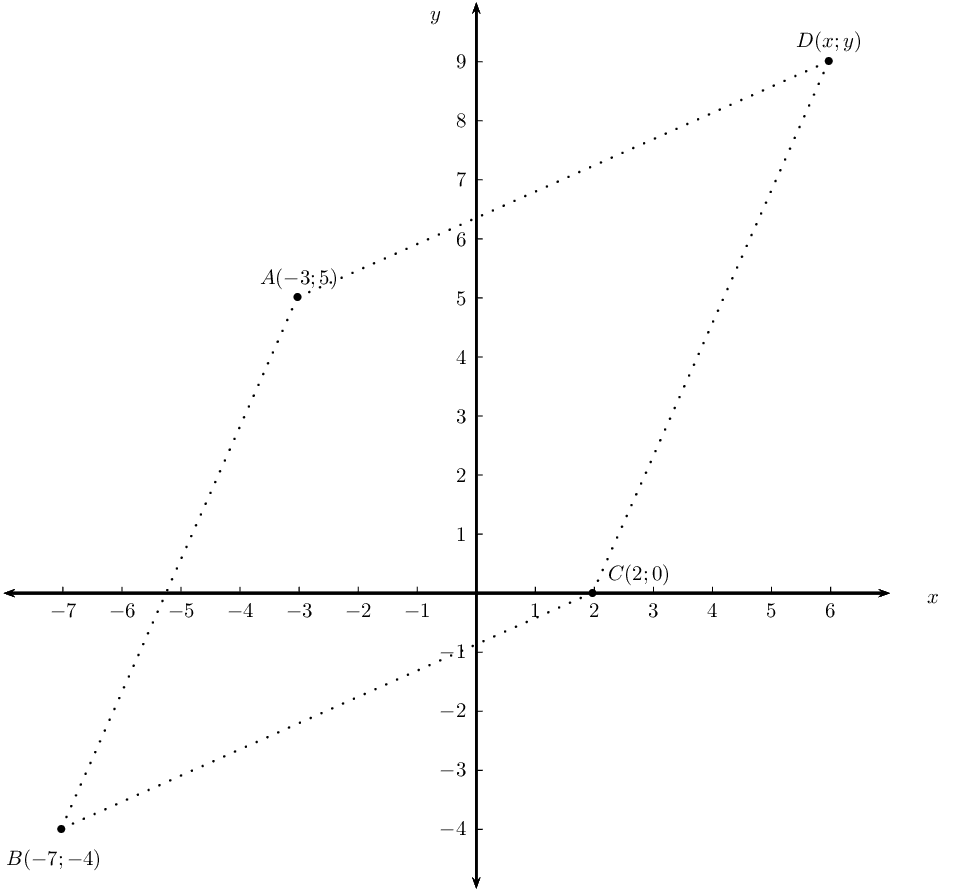Determine the coordinates of $$D$$ if $$ABCD$$ is a parallelogram.

\begin{align*} m_{BC} &= \frac{-4-0}{-7-2} \\ &= \frac{4}{9} \\ \therefore m_{AD} &= \frac{4}{9} \\ \therefore \text{ from } A(-3;5): &\quad 9 \text{ units to the right and } 4 \text{ units up} \end{align*}

Prove that $$ABCD$$ is a rhombus.

\begin{align*} AB &= \sqrt{(x_2 - x_1)^2 + (y_2 - y_1)^2} \\ &= \sqrt{(-7-(-3))^2 + (-4-5)^2} \\ &= \sqrt{(-4)^2 + (-9)^2} \\ &= \sqrt{16 + 81} \\ &= \sqrt{97} \\ AD &= \sqrt{(x_2 - x_1)^2 + (y_2 - y_1)^2} \\ &= \sqrt{(-3-6)^2 + (5-9)^2} \\ &= \sqrt{(-9)^2 + (-4)^2} \\ &= \sqrt{81 + 16} \\ &= \sqrt{97} \\ \therefore AB &= AD \\ \therefore \text{ parallelogram } ABCD &\text{ is a rhombus (adj. sides equal ) } \end{align*}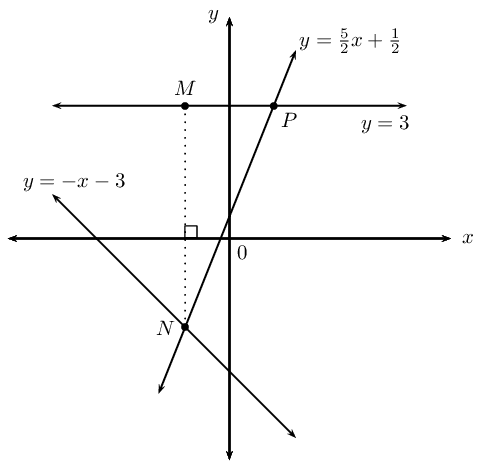Consider the sketch above, with the following lines shown:

$$y = -x -3$$

$$y = 3$$

$$y = \frac{5}{2}x + \frac{1}{2}$$

Determine the coordinates of the point $$N$$.

\begin{align*} \frac{5}{2}x + \frac{1}{2} &= -x - 3 \\ \frac{5}{2}x + x &= - 3 - \frac{1}{2} \\ \frac{7}{2}x &= -\frac{7}{2} \\ \therefore x &= -1 \\ \therefore y &= -(-1) - 3 \\ &= -2 \\ \therefore &N\left( -1;-2 \right) \end{align*}

Determine the coordinates of the point $$P$$.

\begin{align*} y &= \frac{5}{2}x + \frac{1}{2} \\ y &= \frac{5}{2}(3) + \frac{1}{2} \\ &= 8 \\ \therefore &P\left( 8;3 \right) \end{align*}

Determine the equation of the vertical line $$MN$$.

$$x = -1$$

Determine the length of the vertical line $$MN$$.

\begin{align*} MN &= 2 + 3 \\ &= \text{5}\text{ units} \end{align*}

Find $$M\hat{N}P$$.

\begin{align*} \text{Let } M\hat{P}N &= \theta \\ y &= \frac{5}{2}x + \frac{1}{2}\\ \tan \theta &= m \\ \tan \theta &= \frac{5}{2} \\ \therefore \theta &= \text{68,2}\text{°} \\ \text{In } \triangle MNP \quad M\hat{N}P &= \text{180}\text{°} - \text{90}\text{°} - \text{68,2}\text{°} \\ \therefore M\hat{N}P &= \text{21,8}\text{°} \end{align*}

Determine the equation of the line parallel to $$NP$$ and passing through the point $$M$$.

\begin{align*} m &= \frac{5}{2} \\ y &=mx +c \\ y &=\frac{5}{2}x +c \\ \text{Subst. } M(-1;3): \quad 3 &= \frac{5}{2}(-1) + c \\ \therefore c &= 3 + \frac{5}{2} \\ &= \frac{11}{2} \\ \therefore y &=\frac{5}{2}x + \frac{11}{2} \end{align*}

The following points are given: $$A(-2;3)$$, $$B(2;4)$$, $$C(3;0)$$.

Plot the points on the Cartesian plane.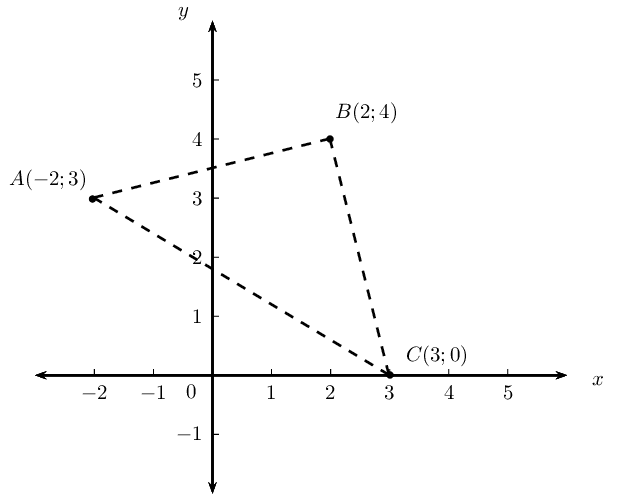Prove that $$\triangle ABC$$ is a right-angled isosceles triangle.

\begin{align*} BC &= \sqrt{(x_2 - x_1)^2 + (y_2 - y_1)^2} \\ &= \sqrt{(3-2)^2 + (0-4)^2} \\ &= \sqrt{(1)^2 + (4)^2} \\ &= \sqrt{1 + 16} \\ &= \sqrt{17} \\ AB &= \sqrt{(x_2 - x_1)^2 + (y_2 - y_1)^2} \\ &= \sqrt{(2+2)^2 + (4-3)^2} \\ &= \sqrt{(4)^2 + (1)^2} \\ &= \sqrt{16 + 1} \\ &= \sqrt{17} \\ \therefore BC &= AB \\ \therefore \triangle ABC &\text{ is isosceles triangle } \\ AC &= \sqrt{(x_2 - x_1)^2 + (y_2 - y_1)^2} \\ &= \sqrt{(3+2)^2 + (0-3)^2} \\ &= \sqrt{(5)^2 + (-3)^2} \\ &= \sqrt{25+9} \\ &= \sqrt{34} \\ \text{And } BC^2 + AB^2 &= 17 + 17 \\ &= 34 \\ &= AC^2 \\ \therefore \triangle ABC &\text{ is right-angled triangle} \end{align*}

Determine the equation of the line $$AB$$.

\begin{align*} m &= \frac{y_2-y_1}{x_2-x_1} \\ &= \frac{4-3}{2+2} \\ &= \frac{1}{4} \\ y &=mx +c \\ y &=\frac{1}{4}x +c \\ \text{Subst. } M(-2;3): \quad 3 &= \frac{1}{4}(-2) + c \\ \therefore c &= \frac{7}{2} \\ \therefore y &=\frac{1}{4}x + \frac{7}{2} \end{align*}

Determine the coordinates of $$D$$ if $$ABCD$$ is a square.

$$D(-1;-1)$$

Determine the coordinates of $$E$$, the mid-point of $$BC$$.

\begin{align*} E(x;y) &= \left( \frac{x_1 + x_2}{2}; \frac{y_1 + y_2}{2} \right) \\ &= \left( \frac{2+3}{2}; \frac{4+0}{2} \right) \\ &= \left( \frac{5}{2}; 2 \right) \end{align*}

Given points $$S(2;5)$$, $$T(-3;-4)$$ and $$V(4;-2)$$.

Determine the equation of the line $$ST$$.

\begin{align*} m &= \frac{y_2-y_1}{x_2-x_1} \\ &= \frac{-4-5}{-3-2} \\ &= \frac{-9}{-6} \\ &= \frac{3}{2} \\ y &=mx +c \\ y &=\frac{3}{2}x +c \\ \text{Subst. } M(2;5): \quad 5 &= \frac{3}{2}(2) + c \\ \therefore c &= 2\\ \therefore y &=\frac{3}{2}x + 2 \end{align*}

Determine the size of $$T\hat{S}V$$.

\begin{align*} m_{ST} &= \frac{3}{2} \\ \tan \beta &= \frac{3}{2} \\ \therefore \beta &= \tan^{-1} \left( \frac{3}{2} \right) \\ &= \text{49,6}\text{°} \\ m_{SV} &= \frac{5+2}{2-4} \\ &= \frac{7}{-2} \\ \tan \theta &= -\frac{7}{2} \\ \therefore \theta &= \tan^{-1} \left( -\frac{7}{2} \right) \\ &= -\text{74,1}\text{°} + \text{180}\text{°} \\ &= \text{105,9}\text{°} \\ \therefore T\hat{S}V &= \text{105,9}\text{°} - \text{56,3}\text{°} \\ &= \text{49,6}\text{°} \end{align*}

Consider triangle $$FGH$$ with vertices $$F(-1;3)$$, $$G(2;1)$$ and $$H(4;4)$$.

Sketch $$\triangle FGH$$ on the Cartesian plane.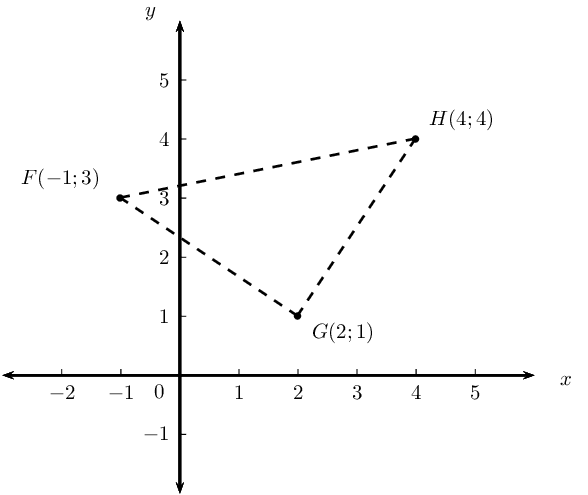Show that $$\triangle FGH$$ is an isosceles triangle.

\begin{align*} FG &= \sqrt{(x_2 - x_1)^2 + (y_2 - y_1)^2} \\ &= \sqrt{(-1-2)^2 + (3-1)^2} \\ &= \sqrt{(-3)^2 + (2)^2} \\ &= \sqrt{9 + 4} \\ &= \sqrt{13} \\ GH &= \sqrt{(x_2 - x_1)^2 + (y_2 - y_1)^2} \\ &= \sqrt{(4-2)^2 + (4-1)^2} \\ &= \sqrt{(2)^2 + (3)^2} \\ &= \sqrt{4 + 9} \\ &= \sqrt{13} \\ \therefore FG &= GH \\ \therefore \triangle FGH &\text{ is isosceles triangle } \end{align*}

Determine the equation of the line $$PQ$$, perpendicular bisector of $$FH$$.

\begin{align*} Q(x;y) &= \left( \frac{x_1 + x_2}{2}; \frac{y_1 + y_2}{2} \right) \\ &= \left( \frac{4-1}{2}; \frac{4+3}{2} \right) \\ &= \left( \frac{3}{2}; \frac{7}{2} \right) \\ m &= \frac{y_2-y_1}{x_2-x_1} \\ &= \frac{4-3}{4+1} \\ &= \frac{1}{5} \\ \therefore m_{\perp} &= -5 \\ y &=mx +c \\ y &=-5x +c \\ \text{Subst. } Q\left( \frac{3}{2}; \frac{7}{2} \right): \quad \frac{7}{2} &= -5 \left( \frac{3}{2} \right) + c \\ \therefore c &= 11 \\ \therefore y &= -5x + 11 \end{align*}

Does $$G$$ lie on the line $$PQ$$?

\begin{align*} y &=-5x + 11 \\ G(2;1) \therefore \text{subst. } x=2: \quad y &= -5 \left( 2 \right) + 11 \\ &= -10 + 11 \\ &= 1 \\ \text{Yes, } G & \text{ lies on PQ } \end{align*}

Determine the equation of the line parallel to $$GH$$ and passing through point $$F$$.

\begin{align*} m_{GH} &= \frac{y_2-y_1}{x_2-x_1} \\ &= \frac{4-1}{4-2} \\ &= \frac{3}{2} \\ y &=mx +c \\ y &=\frac{3}{2}x +c \\ \text{Subst. } F\left( -1;3 \right): \quad 3 &= \frac{3}{2}(-1) + c \\ \therefore c &= \frac{9}{2} \\ \therefore y &= \frac{3}{2}x + \frac{9}{2} \end{align*}

Given the points $$A(-1;5)$$, $$B(5;-3)$$ and $$C(0;-6)$$. $$M$$ is the mid-point of $$AB$$ and $$N$$ is the mid-point of $$AC$$.

Draw a sketch on the Cartesian plane.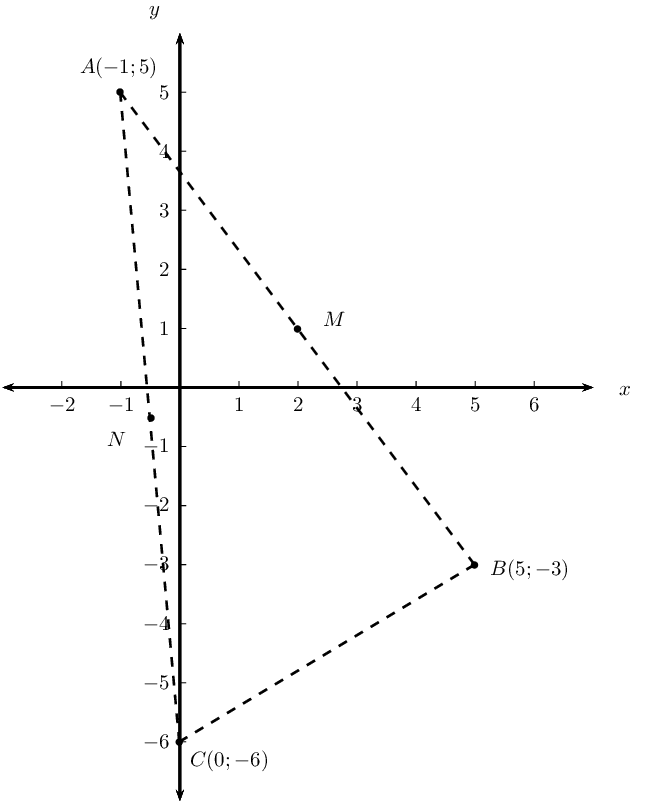Show that the coordinates of $$M$$ and $$N$$ are $$(2;1)$$ and $$(-\frac{1}{2};-\frac{1}{2})$$ respectively.

\begin{align*} M(x;y) &= \left( \frac{x_1 + x_2}{2}; \frac{y_1 + y_2}{2} \right) \\ &= \left( \frac{-1+5}{2}; \frac{5-3}{2} \right) \\ &= \left( \frac{4}{2}; \frac{2}{2} \right) \\ &= \left( 2; 1 \right) \\ N(x;y) &= \left( \frac{x_1 + x_2}{2}; \frac{y_1 + y_2}{2} \right) \\ &= \left( \frac{-1+0}{2}; \frac{5-6}{2} \right) \\ &= \left( -\frac{1}{2}; -\frac{1}{2} \right) \end{align*}

Use analytical geometry methods to prove the mid-point theorem. (Prove that $$NM \parallel CB$$ and $$NM = \frac{1}{2}CB$$.)

\begin{align*} m_{NM} &= \frac{y_2-y_1}{x_2-x_1} \\ &= \dfrac{1+\frac{1}{2}}{2+\frac{1}{2}} \\ &= \dfrac{\frac{3}{2}}{\frac{5}{2}} \\ &= \frac{3}{5} \\ m_{CB} &= \frac{y_2-y_1}{x_2-x_1} \\ &= \frac{-3+6}{5-0} \\ &= \frac{3}{5} \\ m_{NM} &= m_{CB} \\ \therefore NM &\parallel CB \\ NM &= \sqrt{(x_2 - x_1)^2 + (y_2 - y_1)^2} \\ &= \sqrt{(2+\frac{1}{2})^2 + (1+\frac{1}{2})^2} \\ &= \sqrt{(\frac{5}{2})^2 + (\frac{3}{2})^2} \\ &= \sqrt{\frac{25}{4} + \frac{9}{4}} \\ &= \sqrt{\frac{34}{4}} \\ &= \frac{1}{2}\sqrt{34} \\ CB &= \sqrt{(x_2 - x_1)^2 + (y_2 - y_1)^2} \\ &= \sqrt{(5-0)^2 + (-3+6)^2} \\ &= \sqrt{(5)^2 + (3)^2} \\ &= \sqrt{25 + 9} \\ &= \sqrt{34} \\ \therefore NM &= \frac{1}{2}CB \end{align*}